Monday, September 14, 2015

Andy Warhol's Campbell soup

circularized pop art. Mmm mmm good.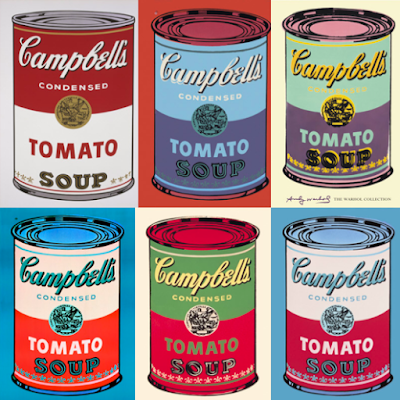input: Andy Warhol's soup cans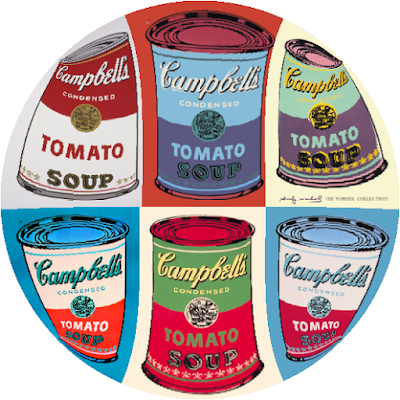Schwarz-Christoffel mappingFG-squircular mapping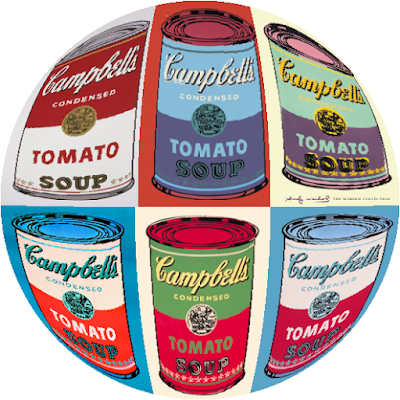elliptical grid mappingShirley-Chiu concentric map

Friday, September 11, 2015

Circular Monopoly

Monopoly is a board game traditionally played on a square board. What happens if we convert the board to a circle?input: Monopoly board game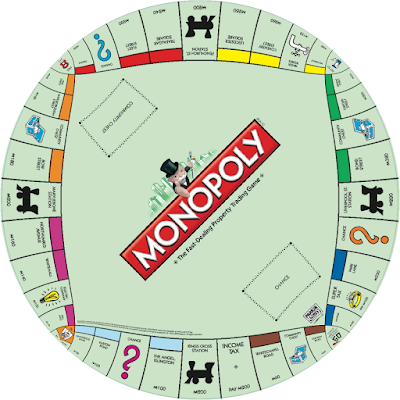Schwarz-Christoffel mappingFG-squircular mappingelliptical grid mappingShirley-Chiu concentric map

Thursday, September 10, 2015

2015 NBA champions

Here is a circular logo of the Golden State Warriors converted into a square.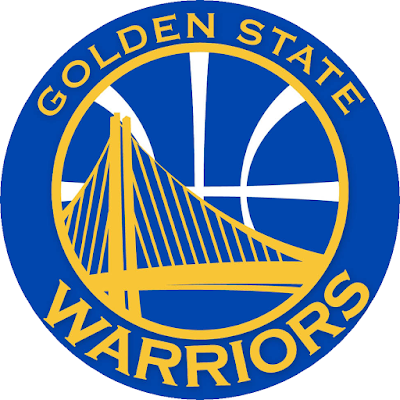input file: Golden State WarriorsSchwarz-Christoffel mappingFG-squircular mappingelliptical grid mappingShirley-Chiu concentric map

Here are results of different square-to-circle mapping algorithms on a bunch of television icons of the 70's. Marcia. Marcia. Marcia!input file: Brady BunchSchwarz-Christoffel conformal mappingelliptical grid mappingFG-squircular mappingShirley-Chiu concentric map

Not to be confused with this "The Brady Bunch Movie" refrigerator magnet I found on ebay!

Wednesday, September 9, 2015

Schwarz-Christoffel mapping

This conformal mapping developed in the 1860s by Hermann Schwarz and Elwin Christoffel can be used to map a circle to a square and vice versa.

The equations for mapping the circular disc to a square region (and vice versa) are:

where z and w are points in the complex plane defined as

z = x + y i
w = u + v i

and

F is the incomplete Legendre elliptic integral of the 1st kind.
cn is a Jacobi elliptic function.
Ke is the complete elliptic integral of the 1st kind with parameter m = ½
(x,y) are coordinates on the square
(u,v) are coordinates on the circular disc

See Section 7 of my paper (page 19) for an explanation of the formulas
http://arxiv.org/ftp/arxiv/papers/1509/1509.06344.pdf

========================================================

Here is an example image of using the Schwarz-Christoffel mapping on a square chessboardSchwarz-Christoffel chessboard

and another on MC Escher's "Circle Limit IV"; i.e. angels and devils inside the Poincare disk.Schwarz-Christoffel for squared Circle Limit IV

Note that the Jacobi cn() function is an even function, so cn(x,k) = cn(-x,k).

Saturday, September 5, 2015

FG-Squircular Mapping

The elliptical grid mapping is by no means the only way to map the square to a circle and vice versa.
In this entry, I shall introduce a disc to square mapping based on the Fernandez-Guasti squircle.

Here are the equations for the mapping.

square to circle:
u = x √(x² + y² - x²y²) / √(x² + y²)
v = y √(x² + y² - x²y²) / √(x² + y²)

circle to square:
x = √½  sgn(uv)/v √(u² + v² - √(u² + v²) √(u² + v² - 4u²v²) )
y = √½  sgn(uv)/u √(u² + v² - √(u² + v²) √(u² + v² - 4u²v²) )

where sgn(z) is the signum function;
(u,v) are circular coordinates in the domain {(u,v) | u² + v² ≤ 1}
(x,y) are square coordinates in the range [-1,1] x [-1,1]

Note: We need to handle zero inputs as a special case.
When input u or v is zero, just set x = u and y = v.
When input x or y is zero, just set u = x and v = y.

The main idea behind this mapping is to map concentric circles inside the circular disc to Fernandez-Guasti squircles inside the square.

See http://squircular.blogspot.com/2015/09/fernandez-guastis-squircle.html for more details on the Fernandez-Guasti squircle.

C++ sample implementation below
--------------------------------------------------------------------------------------------------

// sample code accompanying the paper:
// "Analytical Methods for Squaring the Disc"
// http://arxiv.org/abs/1509.06344

#include <stdio.h>
#include <math.h>

#define epsilon 0.0000001

inline double sgn(double input)
{
double output = 1.0;
if (input < 0.0) {
output = -1.0;
}
return output;
}

// FG-Squircular mapping
// mapping a circular disc to a square region
// input: (u,v) coordinates in the circle
// output: (x,y) coordinates in the square
void fgsDiscToSquare(double u, double v, double& x, double& y)
{
x = u;
y = v;

double u2 = u * u;
double v2 = v * v;
double r2 = u2 + v2;
double uv = u * v;
double fouru2v2 = 4.0 * uv * uv;
double rad = r2 * (r2 - fouru2v2);
double sgnuv = sgn(uv);
double sqrto = sqrt(0.5 * (r2 - sqrt(rad)));

if  (fabs(u) > epsilon)   {
y = sgnuv/u * sqrto;
}

if  (fabs(v) > epsilon) {
x = sgnuv/v * sqrto;
}
}

// FG-Squircular mapping
// mapping a square region to a circular disc
// input: (x,y) coordinates in the square
// output: (u,v) coordinates in the circle
void fgsSquareToDisc(double x, double y, double& u, double& v)
{
u = x;
v = y;

double x2 = x * x;
double y2 = y * y;
double r2 = x2 + y2;
double rad = sqrt( r2 - x2 * y2);

// avoid division by zero if (x,y) is closed to origin
if (r2 < epsilon)   {
return;
}

// This code is amenable to the fast reciprocal sqrt floating point trick
// https://en.wikipedia.org/wiki/Fast_inverse_square_root
double reciprocalSqrt =  1.0/sqrt(r2);

u = x * rad * reciprocalSqrt;
v = y * rad * reciprocalSqrt;
}

int main()
{
double x,y;
double u,v;

fgsSquareToDisc(0.0,-0.789,u,v);
fgsDiscToSquare(u,v,x,y);

printf("%f %f\n",u,v);
printf("%f %f\n",x,y);

fgsDiscToSquare(-0.31415, -0.926535,x,y);
fgsSquareToDisc(x,y,u,v);

printf("%f %f\n",x,y);
printf("%f %f\n",u,v);

return 0;
}

Thursday, September 3, 2015

Fernandez-Guasti's squircle

In 1992, Manuel Fernandez-Guasti introduced a smooth algebraic curve that lies between the circle and the square.

The curve has two parameters: radius r and squareness s

This is a quartic curve (i.e. degree 4) because of the x²y² term.

When s = 0, the FG-squircle is a circle with radius r.
When s = 1, the FG-squircle is a square with side length 2r.

In between, it is a shape that resembles both the circle and the square

Note: For this discussion, we are mainly interested with the curve located in the region
-r ≤ x ≤ r and -r ≤ y ≤ r

This quartic polynomial equation can have values for (x,y) outside this scope, but we can ignore those 4 extraneous regions.

See http://148.206.32.135/mfg/arti/90-94/agrec-ijmest92.pdf
for Fernandez-Guasti's original paper.

for more details.

Squared Corporate Logos

The following logos are circular ones mapped to a square.

Thanks to Seb Przd for inspiring this post

Mapping a Circle to a Square

Philip Nowell (http://mathproofs.blogspot.com/2005/07/mapping-square-to-circle.html)
devised equations for mapping a square to a circle. I will provide the inverse to the mapping below.

For this blog entry, I use a slightly different notation from his equations; i.e.
u = x'
v = y'

(u,v) are circular coordinates in the domain {(u,v) | u² + v² ≤ 1}
(x,y) are square coordinates in the range [-1,1] x [-1,1]

Here are the inverse mapping equations:

x = ½ √( 2 + 2u√2 + u² - v² ) - ½ √( 2 - 2u√2 + u² - v² )
y = ½ √( 2 + 2v√2 - u² + v² ) - ½ √( 2 - 2v√2 - u² + v² )

or more legibly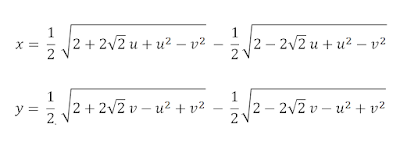The forward equations for the mapping are:

u = x √( 1 - ½ y² )
v = y √( 1 - ½ x² )

Here are some examples of the forward & inverse mappings at work.For a proof/derivation of the inverse equations, see my paper in
arxiv: "Analytical Methods for Squaring the Disc"

In this blog, I shall refer to this mapping as the elliptical grid mapping.  I chose this name because the mapping has a predilection to map a rectilinear grid into a grid of elliptical arcs in the circle.  See Nowell's blog for a discussion and derivation of the elliptical arcs.

Here are more examples of the mapping:Starbucks™ logo converted to a squareAndy Warhol's pop art "Marilyn" as a circle

[ C++ implementation source code below]
------------------------------------------------------------------------------------------

// sample code accompanying the paper:
// "Analytical Methods for Squaring the Disc"
// http://arxiv.org/abs/1509.06344

#include <stdio.h>
#include <math.h>
// Elliptical Grid mapping
// mapping a circular disc to a square region
// input: (u,v) coordinates in the circle
// output: (x,y) coordinates in the square
void ellipticalDiscToSquare(double u, double v, double& x, double& y)
{
double u2 = u * u;
double v2 = v * v;
double twosqrt2 = 2.0 * sqrt(2.0);
double subtermx = 2.0 + u2 - v2;
double subtermy = 2.0 - u2 + v2;
double termx1 = subtermx + u * twosqrt2;
double termx2 = subtermx - u * twosqrt2;
double termy1 = subtermy + v * twosqrt2;
double termy2 = subtermy - v * twosqrt2;
x = 0.5 * sqrt(termx1) - 0.5 * sqrt(termx2);
y = 0.5 * sqrt(termy1) - 0.5 * sqrt(termy2);

}

// Elliptical Grid mapping
// mapping a square region to a circular disc
// input: (x,y) coordinates in the square
// output: (u,v) coordinates in the circle
void ellipticalSquareToDisc(double x, double y, double& u, double& v)
{
u = x * sqrt(1.0 - y*y/2.0);
v = y * sqrt(1.0 - x*x/2.0);
}

int main()
{
double x,y;
double u,v;

ellipticalSquareToDisc(-0.789,0.654,u,v);
ellipticalDiscToSquare(u,v,x,y);

printf("%f %f\n",u,v);
printf("%f %f\n",x,y);

ellipticalDiscToSquare(0.31415, -0.926535,x,y);
ellipticalSquareToDisc(x,y,u,v);

printf("%f %f\n",x,y);
printf("%f %f\n",u,v);

return 0;
}

Simple Stretching: Mapping a circular disc to a square

We can map the circular disc to a square by just linearly stretching each point radially so that the rim of the circle matches the rim of the square.

The equations for the mapping are provided below

where sgn(x) is the signum function.

(u,v) are circular coordinates in the unit disc.
(x,y) are coordinates in the square [-1,1] x [-1,1]

[C++ implementation source code below]
----------------------------------------------------------------------------------

// sample code accompanying the paper:
// "Analytical Methods for Squaring the Disc"
// http://arxiv.org/abs/1509.06344
#include <stdio.h> #include <math.h>// Simple Stretching map // mapping a circular disc to a square region // input: (u,v) coordinates in the circle // output: (x,y) coordinates in the square void stretchDiscToSquare(float u, float v, float& x, float& y) { if ( (fabs(u) < epsilon) || (fabs(v) < epsilon)) { x = u; y = v; return; } float u2 = u * u; float v2 = v * v; float r = sqrt(u2 + v2); // a trick based on Dave Cline's idea // link Peter Shirley's blog if (u2 >= v2) { float sgnu = sgn(u); x = sgnu * r; y = sgnu * r * v / u; } else { float sgnv = sgn(v); x = sgnv * r * u / v; y = sgnv * r; } } // Simple Stretching map // mapping a square region to a circular disc // input: (x,y) coordinates in the square // output: (u,v) coordinates in the circle void stretchSquareToDisc(float x, float y, float& u, float& v) { if ( (fabs(x) < epsilon) || (fabs(y) < epsilon)) { u = x; v = y; return; } float x2 = x*x; float y2 = y*y; float hypothenusSquared = x2 + y2; // code can use fast reciprocal sqrt floating point trick // https://en.wikipedia.org/wiki/Fast_inverse_square_root

Canonical space in disc-to-square mapping

In this blog, I will discuss several ways to map the circular disc to a square and vice versa.  This is the canonical mapping space:

circular domain: {(u,v) | u² + v² ≤ 1}
square range: [-1,1] x [-1,1]

The points (u,v) are in circular disc coordinates
The points (x,y) are in square coordinates

===============================================
Some other relevant links:
1) Stackoverflow.com also discussed the mapping and asked for the inverse.
http://stackoverflow.com/questions/13211595/how-can-i-convert-coordinates-on-a-circle-to-coordinates-on-a-square
http://stackoverflow.com/questions/1621831/how-can-i-convert-coordinates-on-a-square-to-coordinates-on-a-circle

2) Greg Cope has a nice image of a square maze converted to a circular disc.
http://www.algosome.com/articles/maze-generation-depth-first.html

3) Peter Shirley mentioned Dave Cline's trick to simplify his concentric map code
http://psgraphics.blogspot.com/2011/01/improved-code-for-concentric-map.html

4) Stack exchange also has a discussion on this:
http://math.stackexchange.com/questions/440611/geometric-deformations/1662347#1662347